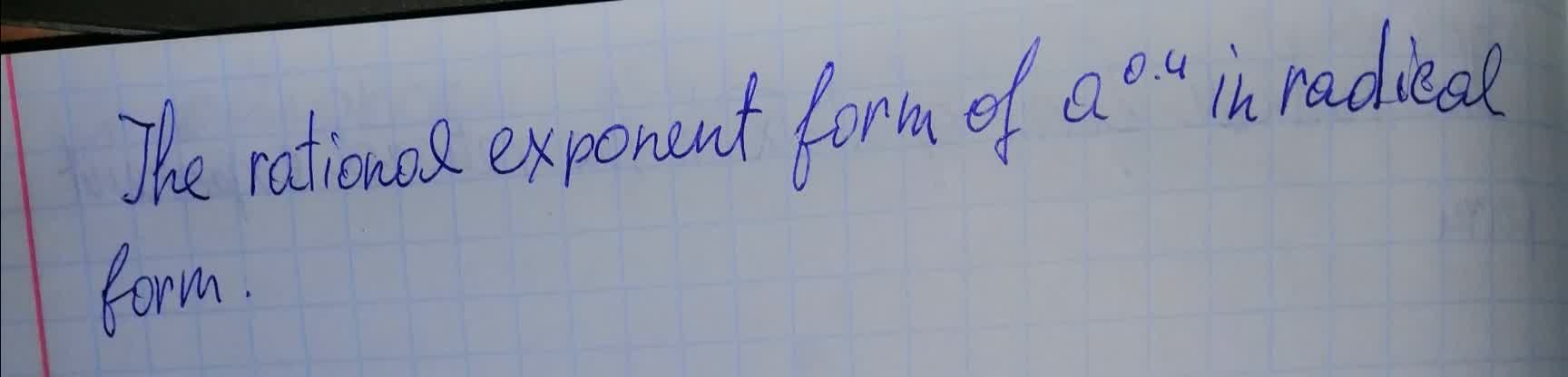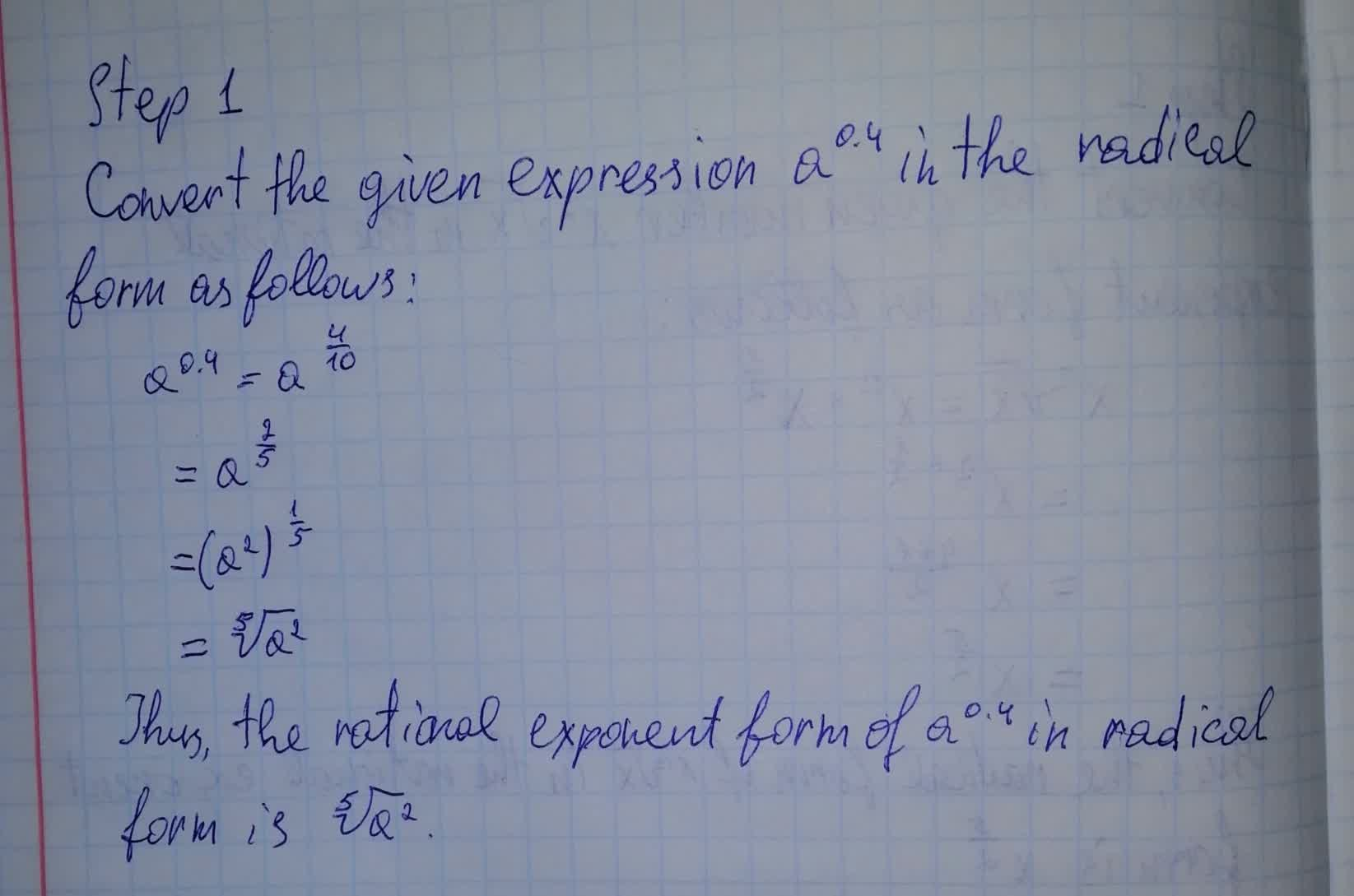# The rational exponent form of a^{0.4} in radical form.remolatg 2021-08-14 Answered
The rational exponent form of ${a}^{0.4}$ in radical form.You can still ask an expert for help

• Questions are typically answered in as fast as 30 minutes

Solve your problem for the price of one coffee

• Math expert for every subject
• Pay only if we can solve itcomentezq
To determine you need###### Not exactly what you’re looking for?Vasquez

Change 0.4 into a fraction.

Multiply by $\frac{10}{10}$ to remove the decimal.

${a}^{\frac{10\cdot 0.4}{10}}$

Multiply 10 by 0.4.

${a}^{\frac{4}{10}}$

Cancel the common factor of 4 and 10

Factor 2 out of 4.

${a}^{\frac{2\left(2\right)}{10}}$

Cancel the common factors.

Factor 2 out of 10.

${a}^{\frac{2\cdot 2}{2\cdot 5}}$

Cancel the common factor.

${a}^{\frac{2\cdot 2}{2\cdot 5}}$

Rewrite the expression.

${a}^{\frac{2}{5}}$

Apply the rule ${x}^{\frac{m}{n}}=\sqrt[n]{{x}^{m}}$ to rewrite the exponentiation as a radical.

$\sqrt{{a}^{2}}$